Function Repository Resource:

# LowestCommonAncestors

Find the lowest common ancestors for pairs of vertices in a graph

Contributed by: Nikolay Murzin
 ResourceFunction["LowestCommonAncestors"][g,v,w] returns a list of the lowest common ancestors of vertices v and w in a graph g. ResourceFunction["LowestCommonAncestors"][g] returns an Association of lowest common ancestors for each distinct pair of vertices in a graph g. ResourceFunction["LowestCommonAncestors"][g,{{v1,w1},{v2,w2},…}] returns the common ancestors for each vertex pair {vi,wi}. ResourceFunction["LowestCommonAncestors"][g,v] returns lowest common ancestors for a vertex v with all other vertices in a graph g.

## Details

Lowest common ancestor is also known as "least", "most recent" or "last" common ancestor.
The lowest common ancestor for a pair of vertices in a graph is their shared ancestor located farthest from the graph root. The graph root is defined as a graph sink of a subgraph induced by the set of common ancestors shared by this pair.
ResourceFunction["LowestCommonAncestors"] works for arbitrary graphs.
LowestCommonAncestors returns a list of vertices for a pair, as there could be zero or more than one lowest common ancestor.
ResourceFunction["LowestCommonAncestors"][g,{v,w}] is equivalent to ResourceFunction["LowestCommonAncestors"][g,v,w].

## Examples

### Basic Examples (4)

Find a list of lowest common ancestors for a pair of vertices in a graph:

 In:=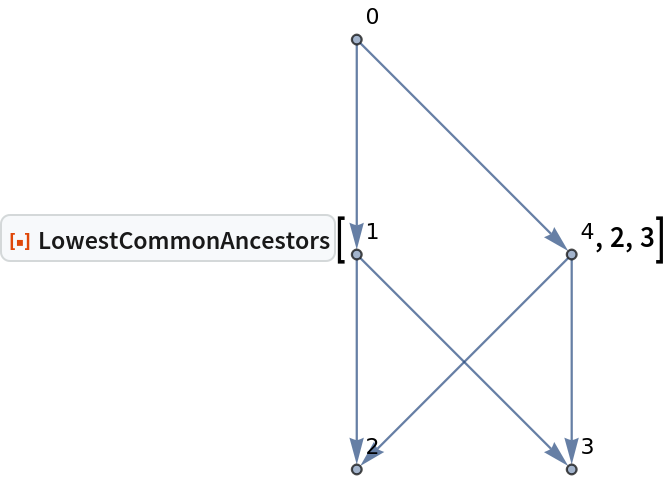Out=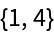Find all lowest common ancestors for a list of pairs of vertices:

 In:=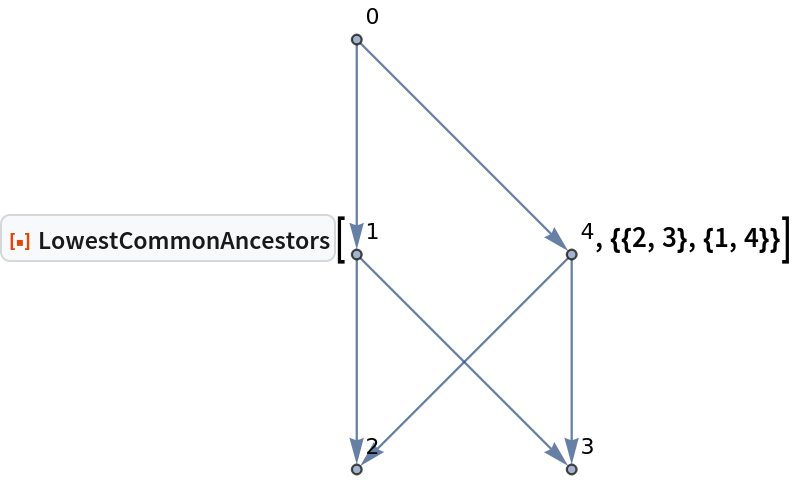Out=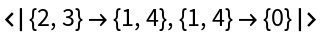Find all lowest common ancestors for all pairs that contain a given vertex:

 In:=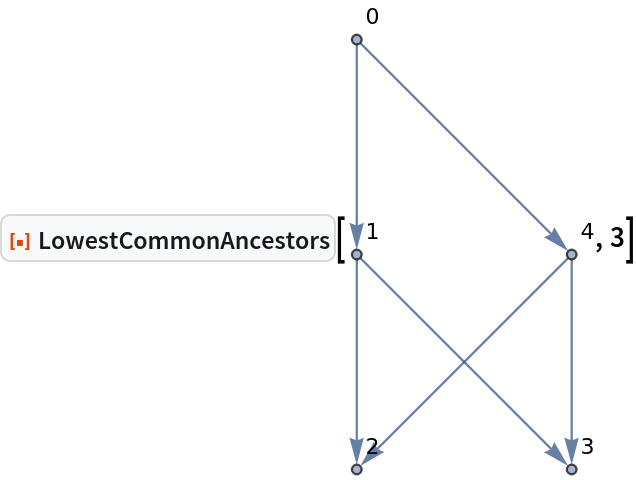Out=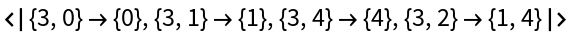Find all lowest common ancestors for every pair of vertices:

 In:=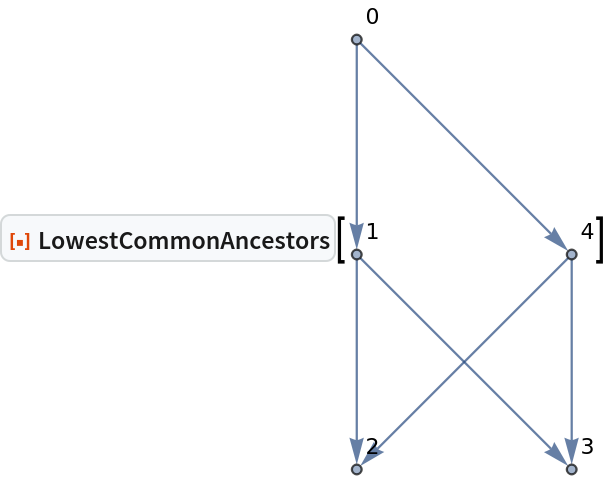Out=In:=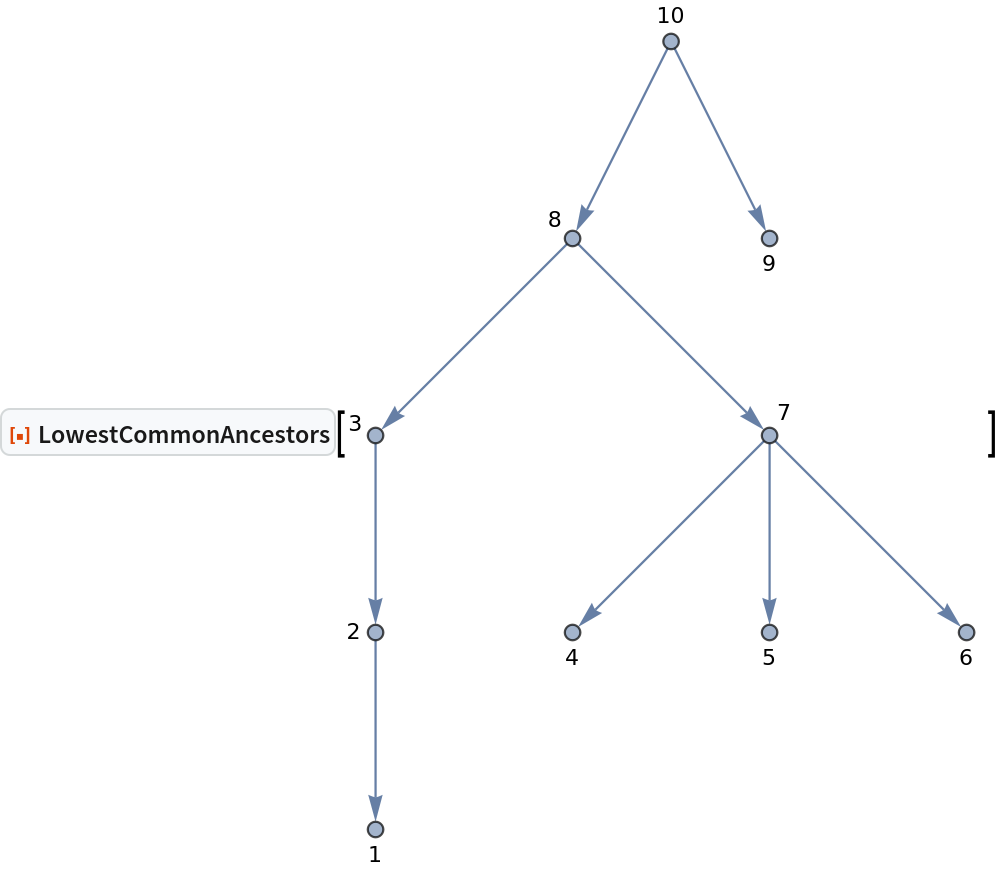Out=### Applications (1)

Measure an average time and spatial distance between pairs of events in a causal graph using longest distance to their lowest common ancestor:

 In:=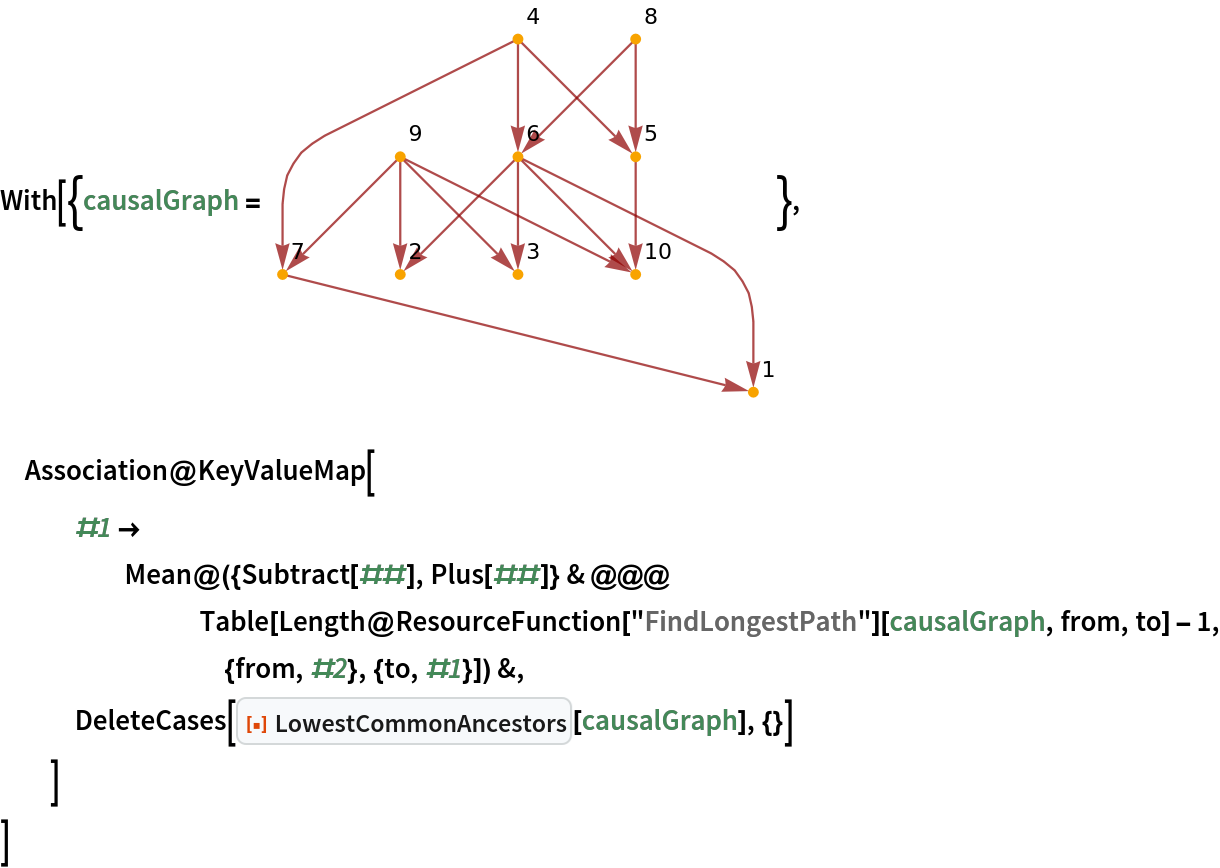Out=## Version History

• 1.0.0 – 01 November 2022